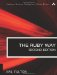# Section 7.16. Adding Intervals to DateTime Values

### 7.15. Comparing Date/Time Values

The Time class conveniently mixes in the Comparable module, so that dates and times may be compared in a straightforward way.

`t0 = Time.local(2000,11,10,22,15)      # 10 Nov 2000 22:15 t1 = Time.local(2000,11,9,23,45)       #  9 Nov 2000 23:45 t2 = Time.local(2000,11,12,8,10)       # 12 Nov 2000  8:10 t3 = Time.local(2000,11,11,10,25)      # 11 Nov 2000 10:25 if t0 < t1 then puts "t0 < t1" end if t1 != t2 then puts "t1 != t2" end if t1 <= t2 then puts "t1 <= t2" end if t3.between?(t1,t2)   puts "t3 is between t1 and t2" end # All four if statements test true`The Ruby Way, Second Edition: Solutions and Techniques in Ruby Programming (2nd Edition)
ISBN: 0672328844
EAN: 2147483647
Year: 2004
Pages: 269
Authors: Hal Fulton

Similar book on Amazon